# Ideal gas law

Values of R Units
8.314472 J·K-1·mol-1
0.082057 L·atm·K-1·mol-1
8.205745 × 10-5 m3·atm·K-1·mol-1
8.314472 L·kPa·K-1·mol-1
8.314472 m3·Pa·K-1·mol-1
62.36367 mmHg·K-1·mol-1
62.36367 Torr·K-1·mol-1
83.14472 L·mbar·K-1·mol-1
10.7316 ft3·psi· °R-1·lb-mol-1
0.73024 ft3·atm·°R-1·lb-mol-1

The ideal gas law is the equation of state of an ideal gas (also known as a perfect gas) that relates its absolute pressure p to its absolute temperature T. Further parameters that enter the equation are the volume V of the container holding the gas and the amount n (in moles) of gas contained in there. The law reads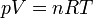$pV = nRT \,$

where R is the molar gas constant, defined as the product of the Boltzmann constant kB and Avogadro's constant NA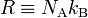$R \equiv N_\mathrm{A} k_\mathrm{B}$

Currently, the most accurate value of R is: 8.314472 ± 0.000015 J·K-1·mol-1.

The law applies to ideal gases which are hypothetical gases that consist of molecules that do not interact, i.e., that move through the container independently of each other. In contrast to what is sometimes stated (see, e.g., Ref.) an ideal gas does not necessarily consist of point particles without internal structure, but may be formed by polyatomic molecules with internal rotational, vibrational, and electronic degrees of freedom. The ideal gas law describes the motion of the centers of mass of the molecules and, indeed, mass centers may be seen as structureless point masses. However, for other properties of ideal gases, such as entropy, the internal structure may play a role.

The ideal gas law is a useful approximation for calculating temperatures, volumes, pressures or amount of substance for many gases over a wide range of values, as long as the temperatures and pressures are far from the values where condensation or sublimation occur.

Real gases deviate from ideal gas behavior because the intermolecular attractive and repulsive forces cause the motions of the molecules to be correlated. The deviation is especially significant at low temperatures or high pressures, i.e., close to condensation. There are many equations of state available for use with real gases, the simplest of which is the van der Waals equation.

##  Historic background

The early work on the behavior of gases began in pre-industrialized Europe in the latter half of the 17th century by Robert Boyle who formulated Boyle's law in 1662 (independently confirmed by Edme Mariotte at about the same time). Their work on air at low pressures established the inverse relationship between pressure and volume, V = constant / p at constant temperature and a fixed amount of air. Boyle's Law is often referred to as the Boyles-Mariotte Law.

In 1699, Guillaume Amontons formulated what is now known as Amontons' law, p = constant / T.

Almost a century later, Jacques Alexandre César Charles experimented with hot-air balloons (around 1780), and additional contributions by John Dalton (1801) and Joseph Louis Gay-Lussac (1808) showed that a sample of gas, at a fixed pressure, increases in volume linearly with the temperature, i.e. V / T is constant.

In 1811, Amedeo Avogadro re-interpreted Gay-Lussac's law of combining volumes to state what is now commonly called Avogadro's law: equal volumes of any two gases at the same temperature and pressure contain the same number of molecules.

In 1834, Benoît Paul Émile Clapeyron combined the work of Boyle, Mariotte, Charles and Gay-Lussac into an equation of state of a perfect (i.e., ideal) gas: PV = RoT where Ro was a gas-dependent constant. In 1845, Victor Regnault cast Clapeyron's perfect gas equation into the familiar ideal gas equation form by applying Avagadro's hypothesis on the volume of one mole of an ideal gas, i.e. PV = nRT.

Sometimes, the ideal gas law is referred to as the Boyle-Gay-Lussac law. However, with hindsight, the Boyle-Gay-Lussac law, Amontons' law and Avogadro's law are all special cases of the ideal gas law.

Extrapolation of the volume/temperature relationship of ideal and many real gases to zero volume crosses the temperature axis at about −273 °C. This temperature is defined as the absolute zero temperature. Since any real gas would liquefy before reaching it, this temperature region remains a theoretical minimum.

##  Statistical mechanics derivation

The statistical mechanics derivation of the ideal gas law provides the most precise insight into the microscopic conditions that a gas must satisfy in order to be called an ideal gas. In the derivation below, we will make the following two assumptions:

1. The molecules constituting the gas are practically independent systems, each pursuing its own motion;
2. Exchange of energy between molecules occasionally takes place, so that the system can achieve a thermal equilibrium.

Starting with the second assumption, we recall from equilibrium statistical mechanics that the canonical partition function is a function of NnNA, V, and T, defined as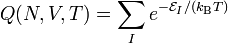$Q(N,V,T) = \sum_I e^{-\mathcal{E}_I/(k_\mathrm{B}T)}$

where$\mathcal{E}_I$ is the I-th energy of the total gas (i.e. the energy of all N molecules). From quantum mechanics follows that a gas in a finite-size container has discrete energies; I is the discrete index labeling the different energies. The sum is over all eigenstates of the energy operator including degeneracies. Further, we recall that the Helmholtz free energy is given by$A = -k_\mathrm{B}T\,\ln Q$. The following expression for the absolute pressure p will be the starting point in the derivation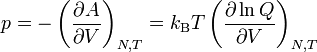$p = -\left(\frac{\partial A}{\partial V}\right)_{N,T} = k_\mathrm{B}T \left( \frac{\partial \ln Q}{\partial V} \right)_{N,T}$

The only approximation (but a very drastic one) that must be made is assumption 1 from above, i.e. that the energies$\mathcal{E}_I$ are sums of one-molecule energies$\varepsilon_i$. These one-molecule energies are those of a single molecule moving by itself in the vessel, an approximation that is common in many branches of physics and known as the independent particle approximation. Thus,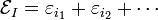$\mathcal{E}_I = \varepsilon_{i_1} + \varepsilon_{i_2} + \cdots$

The total partition function Q will factorize into one-molecule partition functions q given by,$q(N,V,T) = \sum_i e^{-\varepsilon_i/(k_\mathrm{B}T)}$

In absence of interactions Q becomes (assuming that the gas consists of one type of molecules only),$Q = \sum_{i_1,i_2, \ldots} e^{-(\varepsilon_{i_1} + \varepsilon_{i_2} + \cdots)/(k_\mathrm{B}T)} = \sum_{i_1} e^{-\varepsilon_{i_1}/(k_\mathrm{B}T)}\sum_{i_2} e^{-\varepsilon_{i_2}/(k_\mathrm{B}T)}\cdots = q^{N}.$

This form of Q would be correct if the non-interacting gas molecules were non-identical. However, in the early days of quantum mechanics it was discovered that gas molecules of the same type are identical particles, just like electrons, and that a factorial 1/N! must be inserted to avoid overcounting. Later it was shown that this factorial arises from Bose-Einstein statistics (obeyed by the majority of stable molecules). Complete Bose-Einstein statistics itself needs only to be applied at temperatures close to the absolute zero. For higher temperatures Bose-Einstein statistics goes over into Boltzmann statistics, which requires the simple factor 1/N! that we will insert now ad hoc into the expression for Q. In summary, from the additivity of the molecular energies and the application of Boltzmann statistics follows$Q = \frac{q^{N}}{N!}$

The application of Boltzmann statistics is of no consequence to the equation of state, but modifies expressions for other properties of the gas, such as the entropy. The factorization of Q would be exact if (i) the molecules would not interact and if (ii) every molecule had the whole volume V of the container to its disposal, or in other words, if the molecules themselves had zero volume.

Now,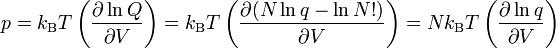$p = k_\mathrm{B}T \left(\frac{\partial \ln Q}{\partial V}\right) = k_\mathrm{B}T \left(\frac{\partial (N\ln q - \ln N!)}{\partial V}\right) = N k_\mathrm{B}T \left( \frac{\partial \ln q}{\partial V}\right)$

where we used the rules ln(a/b) = lna - lnb and lnan = n lna.

It follows from both classical mechanics and quantum mechanics that the molecular energy$\varepsilon_i$ can be exactly separated as$\varepsilon_i = \varepsilon_i^\mathrm{transl} + \varepsilon_i^\mathrm{internal} \quad\Longrightarrow\quad q = q^\mathrm{transl}\; q^\mathrm{internal}$

where$\varepsilon_i^\mathrm{transl}$ is the translational energy of the center of mass of the molecule and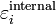$\varepsilon_i^\mathrm{internal}$ is the internal (rotational, vibrational, electronic) energy of the molecule. This factorization of the one-molecule partition function into a translational and an internal factor proceeds in the same way as the factorization of the N-molecule partition function Q into one-molecule partition functions.

The internal energy of the molecule does not depend on the volume V (this is an exact result), but the translational energy does, hence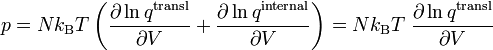$p = N k_\mathrm{B}T\left( \frac{\partial \ln q^\mathrm{transl}}{\partial V} + \frac{\partial \ln q^\mathrm{internal}}{\partial V}\right) = N k_\mathrm{B}T\; \frac{\partial \ln q^\mathrm{transl}}{\partial V}$

The determination of the translational energy of one molecule moving in a box of volume V is one of the few problems in quantum mechanics that can be solved analytically. That is, the energies$\varepsilon_i^\mathrm{transl}$ are known exactly. To a very good approximation, one may replace the sum appearing in qtransl by an integral, finding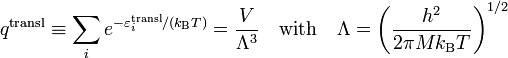$q^\mathrm{transl} \equiv \sum_i e^{-\varepsilon_i^\mathrm{transl}/(k_\mathrm{B}T)} = \frac{V}{\Lambda^3}\quad \hbox{with}\quad \Lambda = \left(\frac{h^2}{2\pi M k_\mathrm{B} T}\right)^{1/2}$

where h is Planck's constant and M is the total mass of the molecule. Note that Λ, the thermal de Broglie wavelength, does not depend on the volume V, so that$p = N k_\mathrm{B}T\left( \frac{\partial (\ln V - 3\ln \Lambda) }{\partial V}\right) = \frac{N k_\mathrm{B}T}{V}$

Here we applied that$\frac{\partial \ln V }{\partial V} = \frac{1}{V}\quad\hbox{and}\quad \frac{\partial \ln \Lambda }{\partial V} = 0$

Using that N = nNA and NAkB = R (see introduction), we have$p\,V = nN_\mathrm{A}\,k_\mathrm{B}\,T = nR\,T$

and that completes the proof of the ideal gas law.